Checkout JEE MAINS 2022 Question Paper Analysis : Checkout JEE MAINS 2022 Question Paper Analysis :

# Linear Programming Problems

What is Linear Programming? A linear programming problem (LPP) which deals with the optimization problem of two linear variables. The function formed using those two linear variables is called objective function.

In our day to day life we encounter many problems forming a linear equation and/or its inequalities. And such as many applications in mathematics involve linear equations/inequalities. Hence those applications based problems which seek to maximize or minimize are optimization problems. In this article we come across solving linear programming problems also.

## What is LPP?

LPP is subject to constraints of linear variables which are non-negative and satisfy the sets of inequalities.

Objective Functions: z = ax + by,

where a and b are to be maximized or minimized depending upon the condition.

Common terminologies used in Linear Programming:

Decision variables: x and y are the decision variables in an objective function.

Non-negative constraints: These are the conditions x, y ≥ 0 are called non negative constraints.

Constraints: The linear inequalities or equations on the variables of a linear programming problem are called constraints.

Optimisation problem: This is a problem which seeks to minimise or maximise a linear function subject to certain constraints as found by a set of linear inequalities. LPP are special types of optimization problems.

Feasible region: It is a region which is common to all the constraints including the non-negative constraints is referred to feasible region.

Infeasible region: It is the region other than the feasible region.

Feasible solutions: Points within and on the boundary of a feasible region denotes feasible solutions of the constraints.

Infeasible solutions: Any point which is outside the feasible region is known as infeasible solution.

Optimal solution: If any point in the feasible region which gives minimum or maximum value of the objective function, it is called an optimal solution.

### Important Results:

Theorem 1: Let R be the feasible region for an LPP and be the objective function. The optimal value of Z must occur at the corner point of the feasible region.

Theorem 2: Let R be the feasible region. If the R is bounded, then the objective function Z, has both maximum and minimum value in region R. And both of these occur at the corner points of R.

If R is unbounded , then a minimum  or maximum value of the objective function may not exist. If it exists, it should come at a corner point of R (by theorem 1)

## Corner Point Method

This is a method of solving LLP. Following are the steps of this method.

1.First find the feasible region of the LLP and find its corner points either by checking or by solving two equations of the lines intersecting at that point.

2.Find objective function Z = ax+by at each corner point. Let M and m represent largest and smallest values at these points respectively.

3.If the feasible region is bounded, M and m are the maximum and minimum values of Z. If the feasible region is unbounded, then

a.M will be the maximum value of Z, if the open half plane determined by ax+by>M which has no point in common with the feasible region. Otherwise Z will not have maximum value.

b.M will be the minimum value of Z, if the open half plane determined by ax+by<m which has no point in common with the feasible region. Otherwise Z will not have minimum value.

## Linear Programming Applications

The most important applications of the linear programming in the following areas:

Manufacturing Problems

These problems are related to industry problems such as some industry is required to produce the number of units of different products, requiring the fixed manpower, operating hours, labour hour per unit of product etc. Such that maximum profit is produced while selling these products.

Example 1:

A company produces two types of TVs, one of which is black and white, the other colour. The company has the resources to make at most 200 sets a week. Creating a black and white set includes Rs 2700 and Rs 3600 to create a colored set. The business should spend no more than Rs 648000 a week producing TV sets. If it benefits from Rs 525 per set of black and white and Rs 675 per set of colours, How many sets of black/white and colored sets it should produce in order to get maximum profit? Formulate this using LPP.

Solution: Let x and y be the number of black/white and colored tvs respectively

Subject to constraints:

x, y ≥ 0 (Non-negative constraint)

x + y ≤ 200 (Quantity constraints)

2700x+3600y ≤ 648000 (Cost constraints)

Objective function: Z = 525x + 675y (objective is to maximize profit)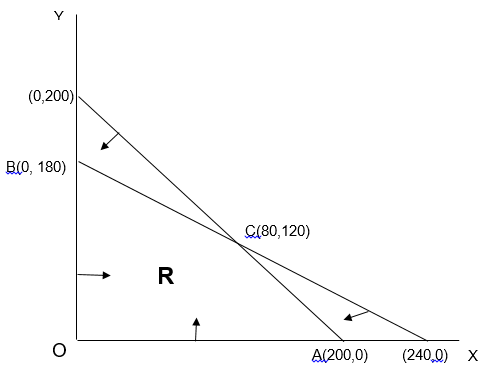Feasible region R are bounded as shown in the figure above.

 Corner Point Objective Function(Z) O(0,0) 525(0) + 675(0) = 0 A(200,0) 525(200) + 675(0) = 105000 C(80,120) 525(80) + 675(120)= 123000 B(0,180) 525(0) + 675(180) = 121500

Thus maximum value of Z occurs at C(80,120) i.e. 123000. So the company should manufacture 80 black/white and 120 colored TV sets to get maximum profit.

Diet Problems

Such kind of problems, a person is required to produce a diet keeping in check of the different nutrients (constraints) required in order to minimize the cost of desired diet.

Example 2:

A health enthusiast wishes to mix two types of foods for his diet, in such a way that vitamin content of the mixture contain at least 10 units of vitamin B and 13 units of vitamin C. Food (F1) contains 1 units/kg of vitamin B and 2 unit/kg of vitamin C. Food (F2) contains 2 unit/kg of vitamin B and contains 1 unit/kg of vitamin C. F1 costs Rs 60/kg and F2 costs Rs 80/kg. Frame his condition of diet plan making linear programming problem in order to minimize the cost of the mixture.

Solution: Let x and y represents the number of units of vitamin B and C respectively.

Subject to constraints:

x, y ≥ 0 (Non-negative constraints)

x + 2y ≥ 10 (Vitamin B constraint)

2x + y ≥ 13 (Vitamin C constraint)

 Resources Food (F1) Food (F2) Requirement Vitamin (B) 1 2 10 Vitamin (C) 2 1 13 Total Cost Rs 60/kg Rs 80/kg

Objective function: Z = 60x + 80y (objective is to minimize cost)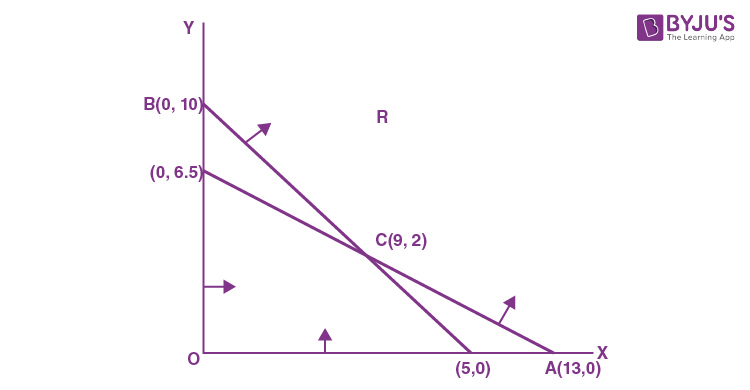Corner Point Objective Function(Z) A(13,0) 60(13) + 80(0) = 780 C(9,2) 60(9) + 80(2) = 700 B(0,10) 60(0) + 80(10) = 800

Feasible region R unbounded as shown in the figure above.

Therefore the minimum value of Z occurs at C(9,2) i.e. 700. So the person should mix 9 kg of food (F1) and 2 kg of food (F2) to obtain minimum cost.

Transportation problems

In these kind of problems, we determine the transportation schedule in order to find the cheapest way of transporting the product from different plants located in different markets.

Example 3:

A jet fuel company has two X and Y depots with 7000 L and 4000 L capacities respectively. The firm is distributing fuel to three jet fuel pumps, D, E and F, respectively in three cities containing 4500L, 3000L, and 3500L. In the following table, the distances (in km) between the depots and jet fuel pumps are given within the following desk:

 Distance (km) From/To X Y D 7 3 E 6 4 F 3 2

If the transport cost of 10 liters of jet fuel is Re 1 per km, how should the distribution be planned to mitigate the transport cost? What’s the lowest cost?

Solution 3: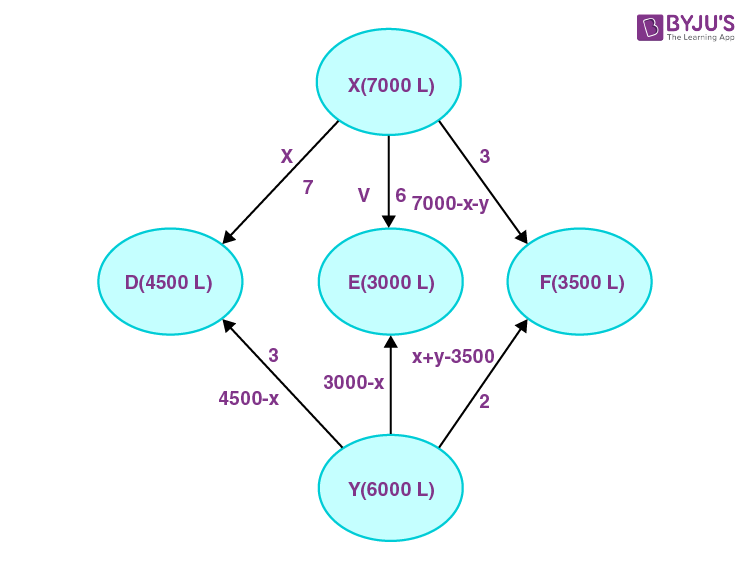Let X supply the fuel pumps, D and E with x and y liters of jet fuel.

So (7000 − x − y) from X to fuel pump F will be delivered. At fuel pump D, the requirement is 4500 L.

The remaining (4500 − x) L will be transferred from fuel pump Y while L is transported from depot X.

Similarly, (3000 − y) L and 3500 − (7000 − x − y) = (x + y − 3500) L will be transported from depot Y to F fuel pump.

Subject to constraints:

x, y ≥ 0

7000 – x – y ≥ 0 (XF constraint)

4500 – x ≥ 0 (YD constraint)

3000 – y ≥ 0 (YE constraint)

x + y – 3500 ≥ 0 (YF constraint)

Cost of transporting 10 L of jet fuel is 1 rupee.

Objective function: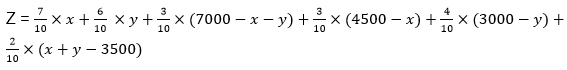The feasible region can be drawn using the subject to constraints condition. The problem can be formulated as follows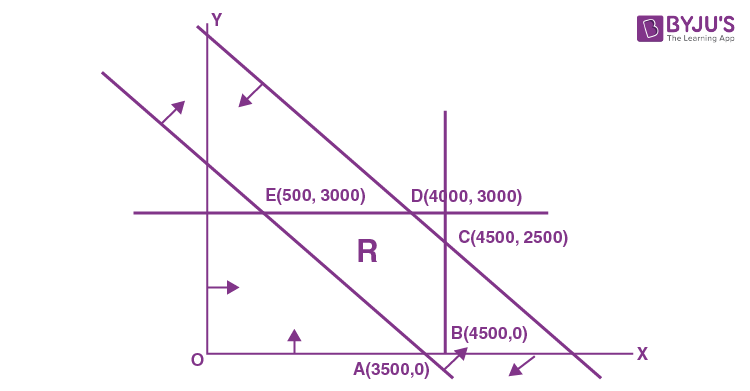Corner Point table:

 Corner Points Z = 0.3x + 0.1y + 3950 A(3500, 0) 5000 B(4500, 0) 5300 C(4500, 2500) 5550 D(4000, 3000) 5450 E(500, 3000) 4400 (Minimum)

The minimum value of Z is 4400 at E(500, 3000).

Thus, the jet fuel supplied from depot A is 500 L, 3000 L, and 3500 L and from depot B is 4000 L, 0 L, and 0 L to fuel pumps D, E, and F respectively.

Therefore, the minimum transportation cost is Rs. 4400.

Example 4: Minimize

$$\begin{array}{l}z=\sum\limits_{j=1}^{n}{{}}\sum\limits_{i=1}^{m}{{{c}_{ij}}\,{{x}_{ij}}}\end{array}$$
subject to
$$\begin{array}{l}\sum\limits_{j=1}^{n}{{{x}_{ij}}\le {{a}_{i}},\ i=1,…….,m}\end{array}$$
,

$$\begin{array}{l}\sum\limits_{i=1}^{m}{{{x}_{ij}}={{b}_{j}},\ j=1,……,n}\end{array}$$
is a (L.P.P.) with number of constraints =

Solution:

Condition (i),

$$\begin{array}{l}i=1,{{x}_{11}}+{{x}_{12}}+{{x}_{13}}+…..+{{x}_{1n}} \\i=2,{{x}_{21}}+{{x}_{22}}+{{x}_{23}}+……+{{x}_{2n}} \\i=3,{{x}_{31}}+{{x}_{32}}+{{x}_{33}}+……+{{x}_{3n}} ……………….. \\i=m,{{x}_{m1}}+{{x}_{m2}}+{{x}_{m3}}+…..{{x}_{mn}}\end{array}$$
→ m constraints

Condition (ii),

$$\begin{array}{l}j=1,\,{{x}_{11}}+{{x}_{21}}+{{x}_{31}}+……+{{x}_{m1}} \\j=2,{{x}_{12}}+{{x}_{22}}+{{x}_{32}}+……+{{x}_{m1}} ……………….. \\j=n,{{x}_{1n}}+{{x}_{2n}}+{{x}_{3n}}+……+{{x}_{mn}}\to n\end{array}$$
→ n constraints

Total constraints = m + n.

Bayes Theorem Of Probability

Truth Tables And Logical Statements

#### Daily-Linear Programming Problems – Video Lesson### What is a Linear Programming problem?

A linear programming problem (LPP) is a problem that is concerned with finding the optimal value of the given linear function.

### Give three limitations of a linear programming problem.

Some of the main limitations of a linear programming problem (LPP) are as follows:
It is difficult to specify the constraints even after the determination of objective function.
It is not easy to find the objective function mathematically in LPP.
The solutions obtained in LPP may not be integers all the time.

### What is the main purpose of solving linear programming problems?

The main aim of the linear programming problem is to determine the optimal solution.

### What do you mean by constraints in LPP?

The constraints are the limitation or restrictions on the decision variables.

Test your Knowledge on Linear programming problems